Common factors are just the factors that two or more numbers have in common.  (These are also called "common divisors.")

Let's just do one:

Find the common factors of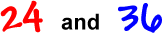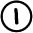List the factors of each: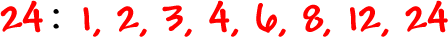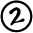Circle the factors that are in both lists: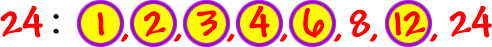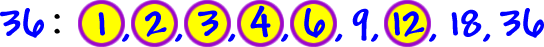The common factors of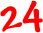and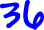are: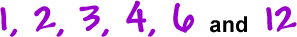Here's another one:

Find the common factors of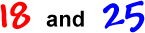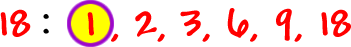The common factor of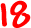andis: